## 20170930

### Presentation: work and energy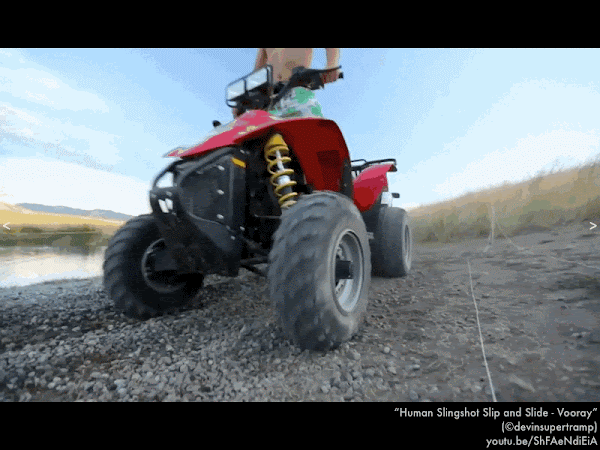Tarp-lined ramp leading down to a pond? Check. All-terrain vehicle? Check. Pulling a cord attached to a raft and rider? Whee! (Video link: "Human Slingshot Slip and Slide - Vooray.")In this presentation we will introduce a new connection between forces and motion, in terms of how forces can do work on or against an object in order to speed up or slow down its motion. This is a new approach to connecting forces and motion, compared to the previous discussion in this course of using Newton's laws to relate how forces on an object result in a net force that may or many not change its motion.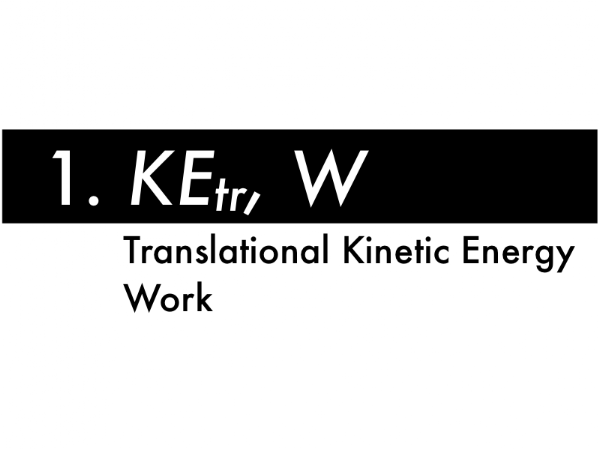First, defining the "amount of motion" of an object in terms of its translational kinetic energy, and then expressing how a force may do work on or against the motion of an object.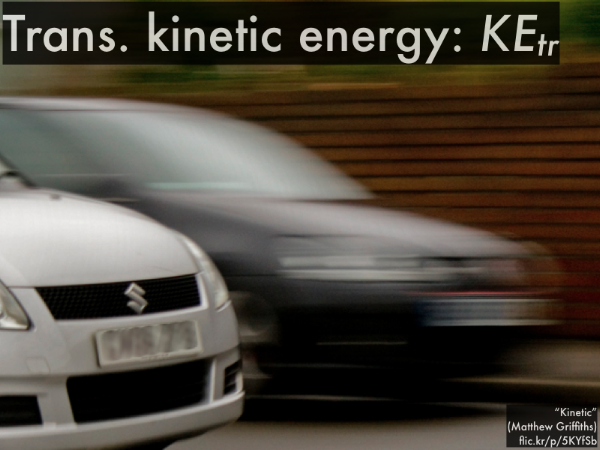Translational kinetic energy KEtr is the energy of motion. (We'll deal with rotational kinetic energy KErot later on.)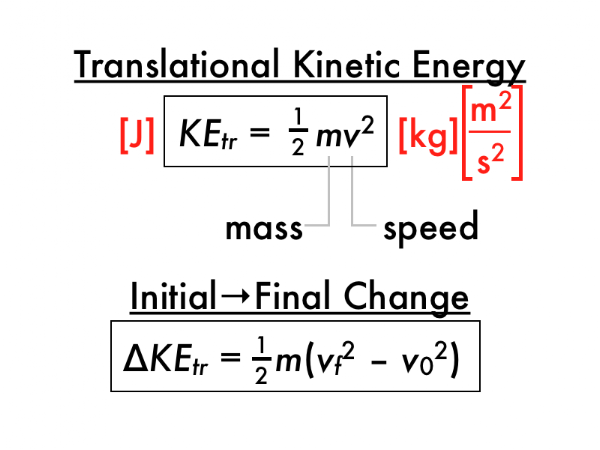Translational kinetic energy KEtr depends on the mass m and the square of the speed v of the object, and the resulting units of kg·m2/s2 are also expressed as joules. A stationary object has no translational kinetic energy, and the faster an object moves, the more translational kinetic energy it has.

Instead of finding out how much translational kinetic energy KEtr an object has, often we are more concerned with its initial-to-final change ∆KEtr, which is the final amount of translational kinetic energy minus the initial amount of translational kinetic energy. Notice how the common factors of (1/2) and mass m (which is presumed to be constant) are pulled out, leaving a "difference of squares" for the final and initial speeds in the parenthesis.In order to change the translational kinetic energy of an object (speeding it up or slowing it down), a force (such as that exerted by the horses) must do a certain amount of work W either on, or against the motion of the object (here the loaded sledge the horses are pulling).Work is accomplished by exerting a force on an object, but in such a way that the object moves through a displacement s (note the unusual use of "s" for a generic ∆x, ∆y, or other direction displacement!), and the "tail-to-tail" angle θ between the force and displacement vectors (when their "bases" are drawn touching together) is anything besides 90°. The units of work are given in N·m, or yet again, joules, so keep in mind that work can be done on or against any mechanical energy form or forms.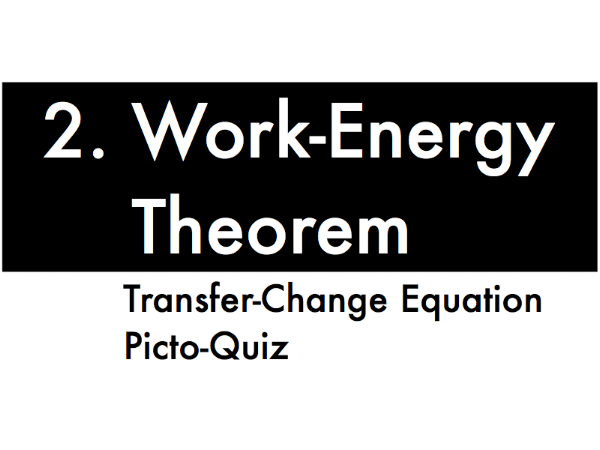Second, let's now explicitly make the connection between the work done by a force acting on or against the motion of an object, and the resulting changes in translational kinetic energy of the object.This is an incomplete form of the total energy conservation equation we will utilize later, but this "work-energy theorem" shows how the transfer of energy (as work is done by a force acting on or against the motion of the object) causes a corresponding change in the translational kinetic energy of the object.

If the force does work on the object (by being exerted along the direction of its motion), then the work will have a positive sign, and the translational kinetic energy of the object will increase, making the sign of the ∆KEtr term positive. Note for this case how the left- and right-hand sides of this equation must have the same positive sign.

If the force does work against the object (by being exerted opposite to the direction of its motion), then the work will have a negative sign, and the translational kinetic energy of the object will decrease, making the sign of the ∆KEtr term negative. Note for this case how the left- and right-hand sides of this equation must have the same negative sign.Squirrel. Catapult! Squirrel catapult! (Note the person behind the sliding glass door, cutting the release cord with a scissors to launch the squirrel.) (Video link: "squirrelcatapult.gif.")

To keep things simple, let's consider a strictly horizontal version of this contraption, which would make the work done by any vertical forces (such as the weight force of Earth on the squirrel) zero, as the angle between these vertical forces and the horizontal direction of motion is 90°.

The bungee cord (and basket) exerts a force on the squirrel directed to the right, along the direction of motion, so the bungee cord does work on the squirrel. As a result, the squirrel picks up speed (starting from rest), and since translational kinetic energy depends on the square of the speed, since speed increases, then the squirrel's translational kinetic energy increases.)

In the work-energy theorem equation:

W = ∆KEtr,

the work will have a positive sign (as work was done on the squirrel by the bungee cords), causing the squirrel's translational kinetic energy to increase (and also have a positive sign), so the +/– signs of both the left-hand side and the right-hand side of the equation are consistent with each other:

(+) = (+).

(If you were to calculate the numerical values for the work done (in J) and the resulting numerical value for the increase in translational kinetic energy (in J), then they would have to be equal to each other (along with having the same sign).
For the catapulted squirrel, the bungee cord force does work __________ the squirrel, which __________ the squirrel's translational kinetic energy.)
(A) on; increases.
(B) against; decreases.
(C) (Unsure/lost/guessing/help!)Note as this car stops, the brakes glow from the heat generated! (Video link: "McLaren SLR review - Top Gear - BBC.")
For the braking car, the brakes do work __________ the car, which __________ the car's translational kinetic energy.
(A) on; increases.
(B) against; decreases.
(C) (Unsure/lost/guessing/help!)Don't blink, or you'll miss Mrs. P-dog in action! (Video link: "110530-1230869-excerpt.")
For Mrs. P-dog being catapulted upwards, the bungee cords do work __________ Mrs. P-dog, while the weight force does work __________ Mrs. P-dog.
(A) on; on.
(B) on; against.
(C) against; on.
(D) against; against.
(E) (Unsure/lost/guessing/help!)

For Mrs. P-dog's translational kinetic energy to be increased while being catapulted upwards, the amount of work from the bungee cords must be __________ the amount of work from the weight force.
(A) less than.
(B) the same as.
(C) greater than.
(D) (Not enough information is given.)
(E) (Unsure/lost/guessing/help!)